# Important CAT FormulasCompetitive exams in India for MBA courses like CAT test a candidates skill on Quantitative Aptitude. The questions in the Quantitative Aptitude section are from various topics like Number Systems, Profit, Loss and Discount, LCM and HCF, Time and Work, Averages, Logarithm, Surds and Indices, Probability, Set Theory & Function, Trigonometry, Permutation & Combination, Co-ordinate Geometry, Mensuration, etc.

Thus, it becomes important that candidates are well versed with the basic formulas required for solving questions with higher accuracy and in the least time possible. Here is a list of important formulae that candidates need to master to crack the Common Admission Test (CAT) successfully.

## Number Systems

• 1 + 2 + 3 + 4 + 5 + … + n = n(n + 1)/2
• (1² + 2² + 3² + ….. + n²) = n ( n + 1 ) (2n + 1) / 6
• (1³ + 2³ + 3³ + ….. + n³) = (n(n + 1)/ 2)²
• Sum of first n odd numbers = n²
• Sum of first n even numbers = n (n + 1)

Mathematical Formulas:

• (a – b)² = (a² + b² – 2ab)
• (a + b)² = (a² + b² + 2ab)
• (a + b)(a – b) = (a² – b²)
• (a + b)² = (a² + b² + 2ab)
• (a + b + c)² = a² + b² + c² + 2(ab + bc + ca)
• (a³ – b³) = (a – b)(a² + ab + b²)
• (a³ + b³) = (a + b)(a² – ab + b²)
• (a³ + b³ + c³ – 3abc) = (a + b + c)(a² + b² + c² – ab – bc – ac)
• When a + b + c = 0, then a³ + b³ + c³ = 3abc
• (a + b)n = an + (nC1)an-1b + (nC2)an-2b² + … + (nCn-1)abn-1 + bn

## Profit, Loss and Discount

• Profit/Gain = (S.P.) – (C.P.)
• Profit % = Profit/(C P)×100
• S P = (100+gain % )/100  ×C P
• C P = 100/(100+gain %)×S P
• Loss = (C.P.) – (S.P.)
• Loss % = Loss/(C.P.)×100
• S P = (100-loss %)/100×C P
• C P = 100/(100-loss %)×S P

## LCM and HCF

LCM × HCF = Product of the Numbers

LCM of Co-prime Numbers = Product Of The Numbers

## Speed, Time and Distance

Distance = Speed x Time

Time = Distance/Speed

Speed= Distance/Time

## Percentages

To find what percentage of x is y: y/x × 100

Increase N by S % = N( 1+ S/100 )

Decrease N by S % = N (1 – S/100)

## Time and Work

If A can do a piece of work in n days, then A’s 1 day’s work = 1/n

If A’s 1 day’s work =1/n, then A can finish the work in n days.

## Averages

Average = (Sum of observations/Number of observations)

## Simple and Compound Interest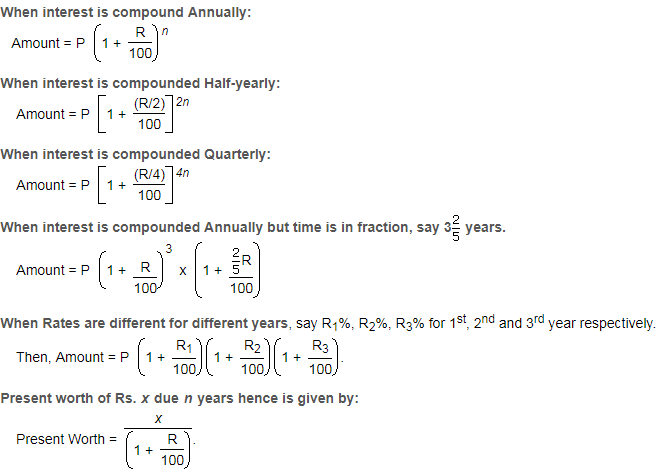## Logarithm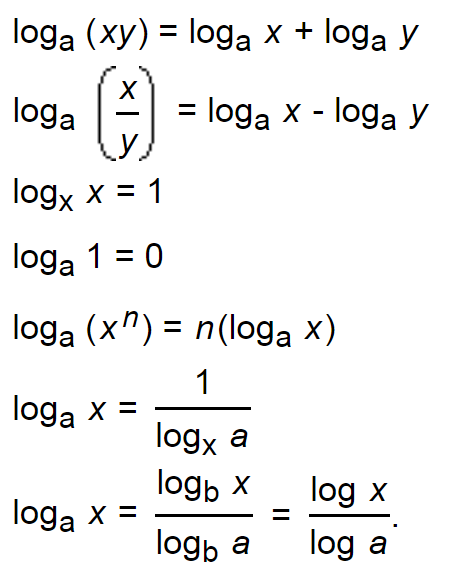## Probability

Sample Space: When we perform an experiment, then the set S of all possible outcomes is called the sample space.

Event: Any subset of a sample space is called an event.

The probability of Occurrence of an Event:

Let S be the sample and let E be an event.

Therefore, P(E) =n(E) / n(S)

## Surds and Indices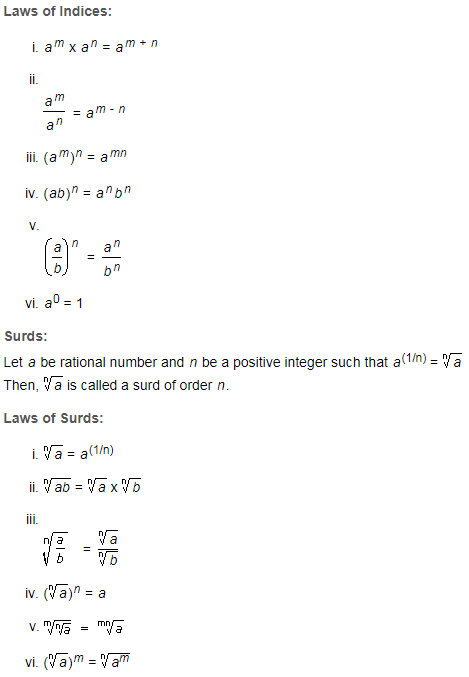## Set Theory & Function

The Demorgan’s Law is the basic and most important formula for sets, which is defined as

(A ∩ B) ‘ = A’ U B’ and (A U B)’ = A’ ∩ B’

The relation R⊂A×AR⊂A×A is said to be called as:

• Reflexive Relation: If a R a ∀∀ a ∈∈ A.
• Symmetric Relation: If aRb, then bRa ∀∀ a, b ∈∈ A.
• Transitive Relation: If aRb, bRc, then aRc ∀∀ a, b, c ∈∈ A.

If any relation R is reflexive, symmetric and transitive in a given set A, then that relation is known as equivalence relation.

## Permutation and Combination

Permutation Formula: A permutation is the choice of r things from a set of n things without replacement. Order matters in permutation.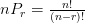Combination Formula: A combination is the choice of r things from a set of n things without replacement. Order does not matter in combination.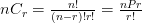## Mixtures and Alligations

Alligation: It is the rule that enables us to find the ratio in which two or more ingredients at the given price must be mixed to produce a mixture of desired price.

Mean Price: The cost of a unit quantity of the mixture is called the mean price.

Rule of Alligation:

If two ingredients are mixed, then

(Quantity of cheaper / Quantity of dearer) = (C.P. of dearer – Mean Price / Mean price – C.P. of cheaper)

## Trigonometry

Trigonometric Identities:

• Sine=Opposite/Hypotenuse
• Secant=Hypotenuse/Adjacent
• Cosine=Adjacent/Hypotenuse
• Tangent=Opposite/Adjacent
• Co−Secant=Hypotenuse/Opposite
• Co−Tangent=Adjacent/Opposite

The reciprocal identities are given as:

• CosecΘ=1/sinΘ
• secΘ=1/cosΘ
• cotΘ=1/tanΘ
• sinΘ=1/CosecΘ
• cosΘ=1/secΘ
• tanΘ=1/cotΘ

## Coordinate Geometry

The Distance Between two Points A and B:

AB² = (Bx – Ax)² + (By – Ay)²

The Midpoint of a Line Joining Two Points

The midpoint of the line joining the points (x1, y1) and (x2, y2) is:

[½(x1 + x2), ½(y1 + y2)]

The Equation of a Line Using One Point and the Gradient

The equation of a line which has gradient m and which passes through the point (x1, y1) is:

y – y1 = m(x – x1)

## Mensuration

Rectangle

Area = lb

Perimeter  = 2(l+b)

Square

Area = a×a

Perimeter = 4a

Triangle

Area =b×h/2 or √s(s-a)(s-b)(s-c)…………….where s=a+b+c/2

Circle

Area =  πr² or πd²/4

Circumference = 2πr  or πd

Area of sector of a circle = (θπr² )/360

Cube

Volume: V = l3

Lateral surface area = 4a2

Surface Area: S = 6s2

Diagonal (d) = √3l

Cuboid

Volume of cuboid: lbh

Total surface area = 2 (lb + bh + hl) or 6l2

Length of diagonal =√(l²+b²+h²)

Stay tuned with BYJU’S to get the latest notifications. Get the detailed CAT exam pattern, cutoff score, eligibility criteria and other CAT exam related details only at BYJU’S.

Also Read: